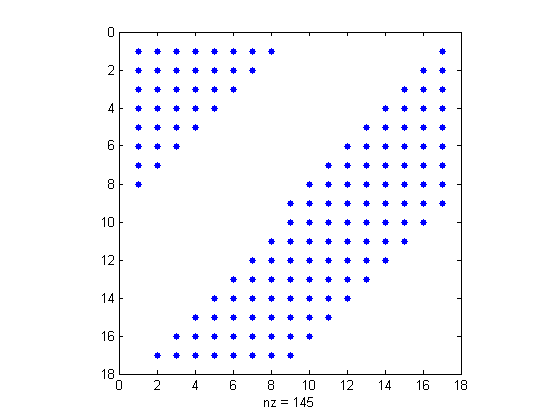## Loren on the Art of MATLABTurn ideas into MATLAB

Note

Loren on the Art of MATLAB has been archived and will not be updated.

# Acting on Specific Elements in a Matrix

Using MATLAB, there are several ways to identify elements from an array for which you wish to perform some action. Depending on how you've chosen the elements, you may either have the list of elements to toss or the list if elements to retain. And you might not have much if any control yourself how the list gets presented to you since the list could be passed to you from another calculation. The lists might be indices, subscripts, or logical arrays (often referred to as masks). Let's look at how you might arrive at such a situation and see what the code looks like to perform one particular action, setting the desired element values to 0.

### Contents

Note: I am not discussing efficiency in this article. It is highly dependent on the number of elements in the original array and how many will be retained or thrown out. This article focuses on specifying what to keep or replace.

### General Setup

Here's the setup for this investigation. I will use a fixed matrix for all the methods and always end up with the same final output. The plan is to show you multiple ways to get the result, since different methods may be appropriate under different circumstances.

A = magic(17);
Result = A;
Result( A < mean(A(:)) ) = 0;

Let's look at the nonzero pattern of Result using spy.

spy(Result)### Method #1 - Using Subscripts of Keepers

Here's a list of the subscripts for the elements to keep unchanged.

[rA,cA] = find(A > (17^2)/2);

Next we convert the subscripts to indices.

Result1 = zeros(size(A));
indices = sub2ind(size(A),rA,cA);
Result1(indices) = A(indices);
isequal(Result, Result1)
ans =
1


Why did I convert subscripts to indices? Let me illustrate with a very small example.

matrix = [ -1 1 0; 2 0 -2; 0 3 -3]
[rows,cols] = find(matrix==0)
matrix =
-1     1     0
2     0    -2
0     3    -3
rows =
3
2
1
cols =
1
2
3


Now let's see what I get if I use the subscripts to address the selected elements:

matrix(rows,cols)
ans =
0     3    -3
2     0    -2
-1     1     0


I get the full matrix back, even though I selected only 3 elements. This definitely surprised me when I first encountered this. What's happening?

MATLAB matches each row element with each column element. matrix([1 2 3],2) returns the elements from rows 1 through 3 in column 1.

matrix(1:3,2)
ans =
1
0
3


### Method #2 - Using Indices of Keepers

Here we used the single output form of find which returns indices instead of subscripts.

indA = find(A > (17^2)/2);
Result2 = zeros(size(A));
Result2(indA) = A(indA);
isequal(Result, Result2)
ans =
1


### Method #3 - Using Logical Keepers

We'll try keeping about half of the elements unchanged.

keepA = (A > (17^2)/2);
Result3 = zeros(size(A));
Result3(keepA) = A(keepA);
isequal(Result, Result3)
ans =
1


keepA is a logical matrix the same size as A. I use logical indexing to populate Result3 with the chosen values from A.

### Method #4 - Subscripts for Elements to Set to Zero

If instead we have a list of candidates to set to 0, we have an easier time since we don't need to start off with a matrix of zeros. Instead we start with a copy of A.

Result4 = A;
[rnotA,cnotA] = find(A <= (17^2)/2);

Convert indices to subscripts, as in method #1.

indices = sub2ind(size(A),rnotA,cnotA);

Now zero out the selected matrix elements.

Result4(indices) = 0;
isequal(Result, Result4)
ans =
1


### Method #5 - Indices for Elements to Set to Zero

If we're instead given indices, we simply skip the step of converting subscripts and follow similar logic to that in method #4.

Result5 = A;
indnotA = find(A <= (17^2)/2);
Result5(indnotA) = 0;
isequal(Result, Result5)
ans =
1


### Method #6 - Using Logical Arrays to Specify Zero Elements

Finally, if we have a mask for the values to set to 0, we simply use it to select and set elements.

Result6 = A;
keepnotA = (A <= (17^2)/2);
Result6(keepnotA) = 0;
isequal(Result, Result6)
ans =
1


### Which Method(s) Do You Prefer?

Which method or methods do you naturally find yourself using? Do you ever invert the logic of your algorithm to fit your way of thinking about addressing the data (the ins or the outs)? Please post your thoughts here. I look forward to seeing them.

Published with MATLAB® 7.6